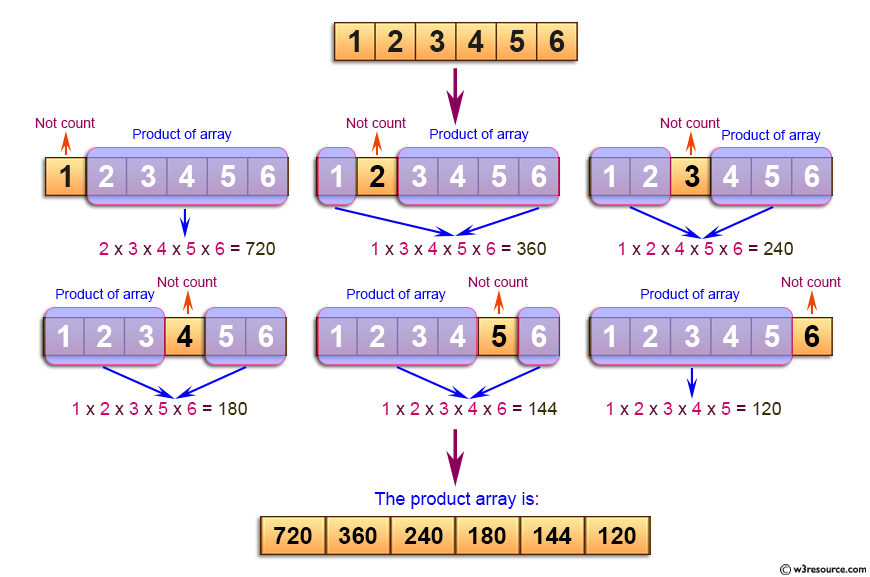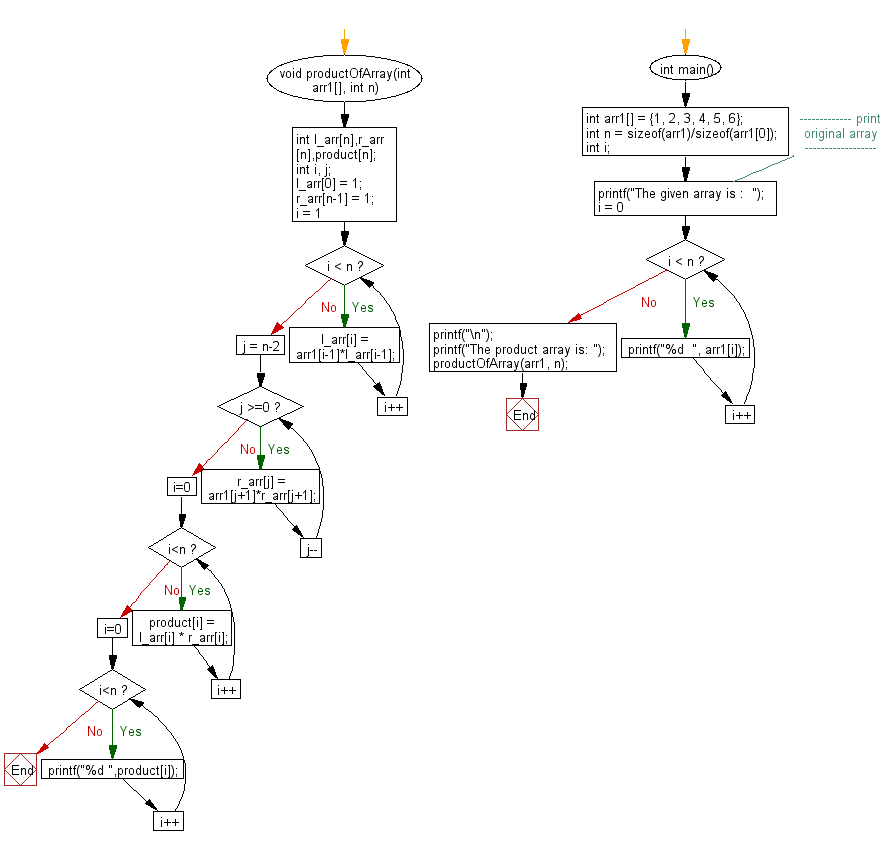﻿ C exercises: Find the product of an array of specified condition - w3resource# C Exercises: Find the product of an array of specified condition

## C Array: Exercise-65 with Solution

Write a program in C to find the product of an array such that product is equal to the product of all the elements of arr[] except arr[i].

Pictorial Presentation:Sample Solution:

C Code:

``````#include <stdio.h>

void productOfArray(int arr1[], int n)
{
int l_arr[n],r_arr[n],product[n];
int i, j;
l_arr = 1;
r_arr[n-1] = 1;
for(i = 1; i < n; i++)
l_arr[i] = arr1[i-1]*l_arr[i-1];
for(j = n-2; j >=0; j--)
r_arr[j] = arr1[j+1]*r_arr[j+1];
for (i=0; i<n; i++)
product[i] = l_arr[i] * r_arr[i];
for (i=0; i<n; i++)
printf("%d ",product[i]);
}

int main()
{
int arr1[] = {1, 2, 3, 4, 5, 6};
int n = sizeof(arr1)/sizeof(arr1);
int i;
//------------- print original array ------------------
printf("The given array is :  ");
for(i = 0; i < n; i++)
{
printf("%d  ", arr1[i]);
}
printf("\n");
//------------------------------------------------------

printf("The product array is: ");
productOfArray(arr1, n);
}
```
```

Sample Output:

```The given array is :  1  2  3  4  5  6
The product array is: 720 360 240 180 144 120
```

Flowchart:C Programming Code Editor:

Improve this sample solution and post your code through Disqus.

What is the difficulty level of this exercise?

﻿

## C Programming: Tips of the Day

Where is the C auto keyword used?

auto is a modifier like static. It defines the storage class of a variable. However, since the default for local variables is auto, you don't normally need to manually specify it.

Ref : https://bit.ly/3yzwC9r